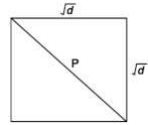# GATE Solved Paper 2017-19 - GATE 2018

• A

πd• B

πd2• C

πd2/4• D

πd/2• Option : D
• Explanation :Area of square = d
Side one side of square = √d
Diagonal of square = √(d+d) = √(2d)
Area of circle = πr2
= π(√(2d)/2)2
= π(d/2)
= (1/2)πd

• A

meandering• B

timely• C

consistent• D

systematic• Option : A
• Explanation :
Meandering: mean is wandering aimlessly/indirect. Consistent is acting or done in the same way over time

• A

3047• B

6047• C

7987• D

63847• Option : C
• Explanation :
From the given number is divided by either by 20 or 42 or by 76 K*LCM (20,42,76) + constant difference = 7980 K + 7 is natural number . So the Ans is 7987

• A

Their, they’re there• B

They’re, their, there• C

There, their, they’re• D

They’re, there, there• Option : B
• Explanation :
In this question They’re used for pointing group. There is pointing people. There is used for place.

• A

2880• B

1440• C

720• D

0• Option : B
• Explanation :